Latest Banking jobs   »   Quantitative Aptitude Quiz For RBI Attendant...

# Quantitative Aptitude Quiz For RBI Attendant 2021- 12th March

Q1. A man invested Rs. 9600 in two equal parts on SI for T years and (T + 2) years at the rates of 12.5% p.a and 16% p.a respectively. If the man got a total interest of Rs. 4272, then find the value of T.
(a) 1 years
(b) 3 years
(c) 1.5 years
(d) 2.5 years
(e) 2 years

Q2. Veer invested Rs. P at the rate of 15% p.a and Rs. (P + 800) at the rate of 8.5% p.a. If veer gets a total simple Interest of Rs. 4836 after two years, then find the value of (P + 800).
(a) 9600 Rs.
(b) 10400 Rs.
(c) 10800 Rs.
(d) 11800 Rs.
(e) 12600 Rs.

Q3. A man invested Rs. 8500 on SI at the rate of R% per annum. If ratio between rate of interest and time period is 4 : 1 and total interest received is Rs. 1360 then find the time for which the man invested his sum?
(a) 1.5 years
(b) 2.5 years
(c) 3 years
(d) 4 years
(e) 2 years

Q4. Mr. Adarsh invested certain principal sum on SI and got 34% more amount after four years. Find the rate for which Adarsh invested his sum?
(a) 12.5 %
(b) 8.5 %
(c) 10 %
(d) 8 %
(e) 12%

Q5. Abhishek borrowed Rs. 12600 from Satish on SI at the rate of 15% per annum. If Abhishek paid back Rs. 6380 at the end of second year, find how much amount Abhishek will pay to Satish at the end of next two years?
(a) 12800 Rs.
(b) 10500 Rs.
(c) 13000 Rs.
(d) 13800 Rs.
(e) 14600 Rs.

Q6. Ritu invested Rs. 16200 at the rate of R% per annum on SI. If she got a total amount of Rs 25920 at the end of four years, then what amount will Ritu get after two years, if rate of interest increased by 5%?
(a) 22800 Rs.
(b) 22000 Rs.
(c) 22680 Rs.
(d) 24000 Rs.
(e) 21500 Rs.

Q7. A man invested a sum in a scheme ‘P’ which offer 10% C.I.P.A. for 3 years and earn Rs. 1655. Find the interest earn by man, if he invested total amount he get from scheme ‘P’, in scheme Q which offer 8% S.I.P.A for 5 years.
(a) Rs.4317
(b) Rs.1655
(c) Rs.1331
(d) Rs.2662
(e) Rs.2000

Q8. A sum becomes Rs. 4840 in two years and Rs. 5856.4 in four years when compounded annually. What would be the amount if same sum invested at 15% S.I. for 3 years (in Rs.) ?
(a) 5600
(b) 5000
(c) 6400
(d) 6200
(e) 5800

Q9. Difference of the interest received when a sum is invested at 15% p.a. at SI for two years and the interest received when that sum is invested at 20% p.a. for one year compounded half yearly is Rs 432, find the sum?
(a) Rs. 5400
(b) Rs. 5000
(c) Rs. 4500
(d) Rs. 4000
(e) Rs. 4800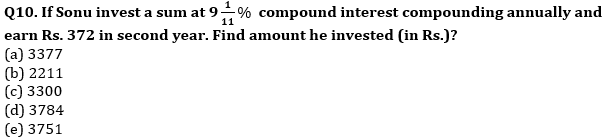Q11. An amount is invested at S.I. for three years at rate of 5% and S.I. received on that amount is Rs. 180. If the same amount is invested for two years at 10% C.I. for first year & R% C.I. for 2nd year & total C.I. received in two years is Rs. 318 then find value of R ?
(a) 15%
(b) 12%
(c) 22%
(d) 18%
(e) 25%

Q12. Veer invested an amount on simple interest, and it becomes two times of itself in 10 years. If Veer invested Rs. 1600 at the same rate of interest on CI, then find amount will get by Veer after three years (in Rs.)?
(a) 2100.6
(b) 2184.6
(c) 2196.6
(d) 2129.6
(e) 2164.6

Q13. A man invests a sum of 3600 in two part one at rate of 5% and other at rate of 7% and get total interest 204 as SI at the end of year. Find what part was invested at 7% rate.
(a) 1200
(b) 2400
(c) 1800
(d) 3000
(e) 600

Q14. A man invests 2500 Rs at 20% Compounded annually. Find the difference between interest earned in second year and third year.
(a) 100
(b) 220
(c) 20
(d) 120
(e) 60

Q15. Difference of the interest received when a sum is invested at 15% p.a. at SI for two years and the interest received when that sum is invested at 20% p.a. for one year compounded half yearly is Rs 432, find the sum?
(a) Rs. 5400
(b) Rs. 5000
(c) Rs. 4500
(d) Rs. 4000
(e) Rs. 4800

Practice More Questions of Quantitative Aptitude for Competitive Exams:

###### 40 Days Study Plan for RBI Office Attendant Exam 2021

Solutions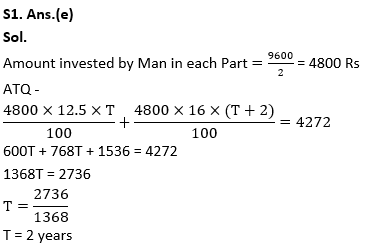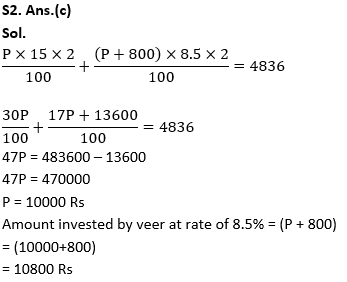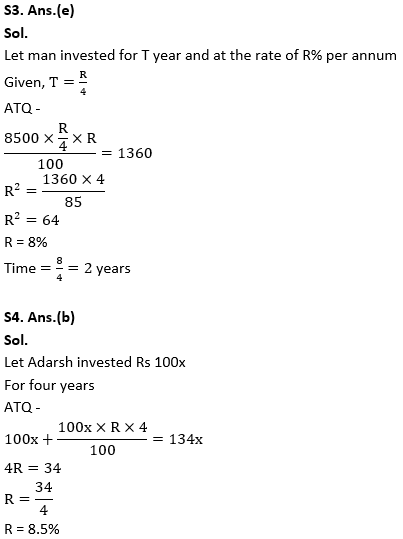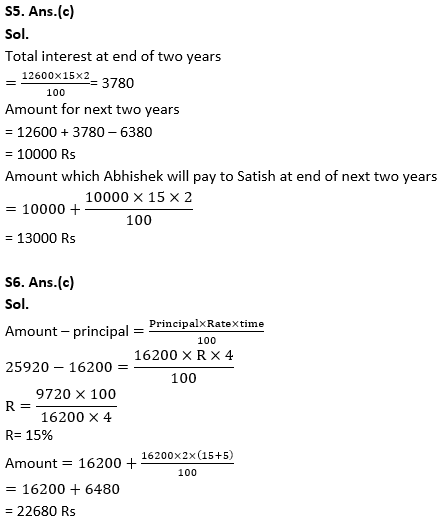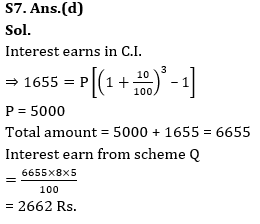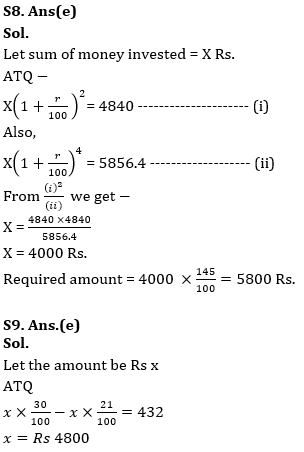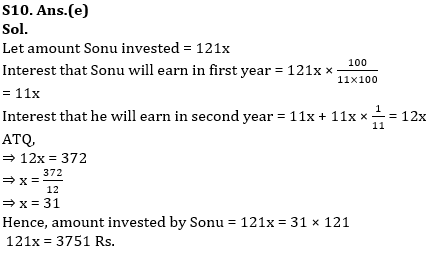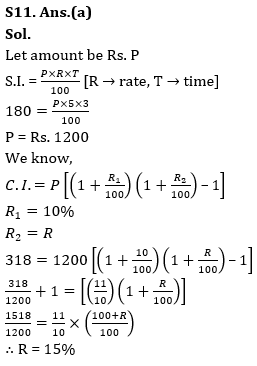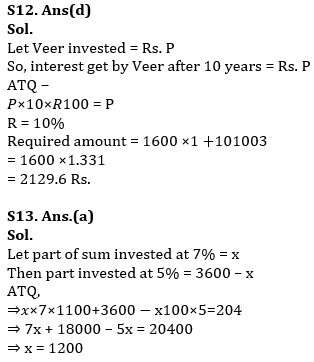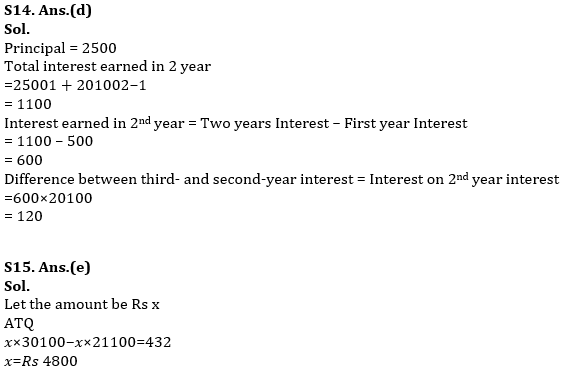Practice with Online Test Series for RBI Attendant 2021:×

Thank You, Your details have been submitted we will get back to you.Join India's largest learning destination

What You Will get ?

•Job Alerts
•Daily Quizzes
•Subject-Wise Quizzes
•Current Affairs
•Previous year question papers
•Doubt Solving session

ORJoin India's largest learning destination

What You Will get ?

•Job Alerts
•Daily Quizzes
•Subject-Wise Quizzes
•Current Affairs
•Previous year question papers
•Doubt Solving session

ORJoin India's largest learning destination

What You Will get ?

•Job Alerts
•Daily Quizzes
•Subject-Wise Quizzes
•Current Affairs
•Previous year question papers
•Doubt Solving session

Enter the email address associated with your account, and we'll email you an OTP to verify it's you.Join India's largest learning destination

What You Will get ?

•Job Alerts
•Daily Quizzes
•Subject-Wise Quizzes
•Current Affairs
•Previous year question papers
•Doubt Solving session

Enter OTP

Please enter the OTP sent to
/6

Did not recive OTP?

Resend in 60sJoin India's largest learning destination

What You Will get ?

•Job Alerts
•Daily Quizzes
•Subject-Wise Quizzes
•Current Affairs
•Previous year question papers
•Doubt Solving sessionJoin India's largest learning destination

What You Will get ?

•Job Alerts
•Daily Quizzes
•Subject-Wise Quizzes
•Current Affairs
•Previous year question papers
•Doubt Solving session

Almost there

+91Join India's largest learning destination

What You Will get ?

•Job Alerts
•Daily Quizzes
•Subject-Wise Quizzes
•Current Affairs
•Previous year question papers
•Doubt Solving session

Enter OTP

Please enter the OTP sent to Edit Number

Did not recive OTP?

Resend 60

By skipping this step you will not recieve any free content avalaible on adda247, also you will miss onto notification and job alerts

Are you sure you want to skip this step?

By skipping this step you will not recieve any free content avalaible on adda247, also you will miss onto notification and job alerts

Are you sure you want to skip this step?